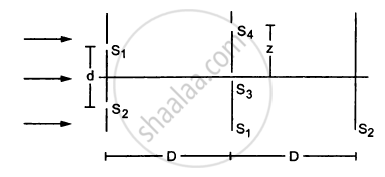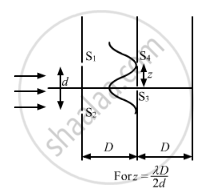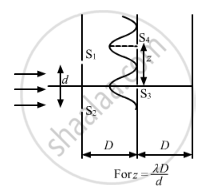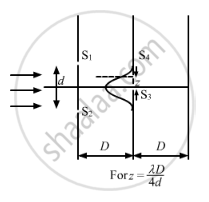Department of Pre-University Education, KarnatakaPUC Karnataka Science Class 12
Advertisement Remove all ads

# Consider the Situation Shown in the Figure. the Two Slits S1 and S2 Placed Symmetrically Around the Central Line Are Illuminated by a Monochromatic Light of Wavelength λ. - Physics

Sum

Consider the situation shown in the figure. The two slits S1 and S2 placed symmetrically around the central line are illuminated by a monochromatic light of wavelength λ. The separation between the slits is d. The light transmitted by the slits falls on a screen ∑1placed at a distance D from the slits. The slit S3 is at the central line and the slit S4 is at a distance z from S3. Another screen ∑2 is placed a further distance D away from ∑1.Find the ratio of the maximum to minimum intensity observed on ∑2 if z is equal to(a) $z = \frac{\lambda D}{2d}$

(b) $\frac{\lambda D}{d}$

(c) $\frac{\lambda D}{4d}$

Advertisement Remove all ads

#### Solution

Given:
Separation between the two slits = d
Wavelength of the light = $\lambda$

Distance of the screen = D

The fringe width (β) is given by $\beta = \frac{\lambda D}{d}$

At S3, the path difference is zero. So, the maximum intensity occurs at amplitude = 2a.

(a) When $z = \frac{D\lambda}{2d}$

The first minima occurs at S4, as shown in figure (a).

With amplitude = 0 on screen ∑2we get

$\frac{l_{max}}{l_{min}} = \frac{\left( 2a + 0 \right)^2}{\left( 2a - 0 \right)^2} = 1$(b) When $z = \frac{D\lambda}{d}$

The first maxima occurs at S4, as shown in the figure.With amplitude = 2a on screen ∑2, we get

$\frac{l_\max}{l_\min} = \frac{\left( 2a + 2a \right)^2}{\left( 2a - 2a \right)^2} = \infty$

(c) When $z = \frac{D\lambda}{4d}$The slit S4 falls at the mid-point of the central maxima and the first minima, as shown in the figure.

Intensity $= \frac{l_\max}{2}$

$\Rightarrow \text{Amplitude }= \sqrt{2}a$

$\therefore \frac{l_\max}{l_\min} = \frac{\left( 2a + \sqrt{2}a \right)^2}{\left( 2a - \sqrt{2}a \right)^2} = 34$

Concept: Refraction of Monochromatic Light
Is there an error in this question or solution?
Advertisement Remove all ads

#### APPEARS IN

HC Verma Class 11, 12 Concepts of Physics 1
Chapter 17 Light Waves
Q 33 | Page 383
Advertisement Remove all ads
Advertisement Remove all ads
Share
Notifications

View all notifications

Forgot password?